# sklearn.cluster.MiniBatchKMeans¶

class sklearn.cluster.MiniBatchKMeans(n_clusters=8, init='k-means++', max_iter=100, batch_size=100, verbose=0, compute_labels=True, random_state=None, tol=0.0, max_no_improvement=10, init_size=None, n_init=3, reassignment_ratio=0.01)[source]

Mini-Batch K-Means clustering

Read more in the User Guide.

Parameters: n_clusters : int, optional, default: 8 The number of clusters to form as well as the number of centroids to generate. init : {‘k-means++’, ‘random’ or an ndarray}, default: ‘k-means++’ Method for initialization, defaults to ‘k-means++’: ‘k-means++’ : selects initial cluster centers for k-mean clustering in a smart way to speed up convergence. See section Notes in k_init for more details. ‘random’: choose k observations (rows) at random from data for the initial centroids. If an ndarray is passed, it should be of shape (n_clusters, n_features) and gives the initial centers. max_iter : int, optional Maximum number of iterations over the complete dataset before stopping independently of any early stopping criterion heuristics. batch_size : int, optional, default: 100 Size of the mini batches. verbose : boolean, optional Verbosity mode. compute_labels : boolean, default=True Compute label assignment and inertia for the complete dataset once the minibatch optimization has converged in fit. random_state : int, RandomState instance or None (default) Determines random number generation for centroid initialization and random reassignment. Use an int to make the randomness deterministic. See Glossary. tol : float, default: 0.0 Control early stopping based on the relative center changes as measured by a smoothed, variance-normalized of the mean center squared position changes. This early stopping heuristics is closer to the one used for the batch variant of the algorithms but induces a slight computational and memory overhead over the inertia heuristic. To disable convergence detection based on normalized center change, set tol to 0.0 (default). max_no_improvement : int, default: 10 Control early stopping based on the consecutive number of mini batches that does not yield an improvement on the smoothed inertia. To disable convergence detection based on inertia, set max_no_improvement to None. init_size : int, optional, default: 3 * batch_size Number of samples to randomly sample for speeding up the initialization (sometimes at the expense of accuracy): the only algorithm is initialized by running a batch KMeans on a random subset of the data. This needs to be larger than n_clusters. n_init : int, default=3 Number of random initializations that are tried. In contrast to KMeans, the algorithm is only run once, using the best of the n_init initializations as measured by inertia. reassignment_ratio : float, default: 0.01 Control the fraction of the maximum number of counts for a center to be reassigned. A higher value means that low count centers are more easily reassigned, which means that the model will take longer to converge, but should converge in a better clustering. cluster_centers_ : array, [n_clusters, n_features] Coordinates of cluster centers labels_ : Labels of each point (if compute_labels is set to True). inertia_ : float The value of the inertia criterion associated with the chosen partition (if compute_labels is set to True). The inertia is defined as the sum of square distances of samples to their nearest neighbor.

KMeans
The classic implementation of the clustering method based on the Lloyd’s algorithm. It consumes the whole set of input data at each iteration.

Notes

Examples

>>> from sklearn.cluster import MiniBatchKMeans
>>> import numpy as np
>>> X = np.array([[1, 2], [1, 4], [1, 0],
...               [4, 2], [4, 0], [4, 4],
...               [4, 5], [0, 1], [2, 2],
...               [3, 2], [5, 5], [1, -1]])
>>> # manually fit on batches
>>> kmeans = MiniBatchKMeans(n_clusters=2,
...         random_state=0,
...         batch_size=6)
>>> kmeans = kmeans.partial_fit(X[0:6,:])
>>> kmeans = kmeans.partial_fit(X[6:12,:])
>>> kmeans.cluster_centers_
array([[1, 1],
[3, 4]])
>>> kmeans.predict([[0, 0], [4, 4]])
array([0, 1], dtype=int32)
>>> # fit on the whole data
>>> kmeans = MiniBatchKMeans(n_clusters=2,
...         random_state=0,
...         batch_size=6,
...         max_iter=10).fit(X)
>>> kmeans.cluster_centers_
array([[3.95918367, 2.40816327],
[1.12195122, 1.3902439 ]])
>>> kmeans.predict([[0, 0], [4, 4]])
array([1, 0], dtype=int32)


Methods

 fit(X[, y, sample_weight]) Compute the centroids on X by chunking it into mini-batches. fit_predict(X[, y, sample_weight]) Compute cluster centers and predict cluster index for each sample. fit_transform(X[, y, sample_weight]) Compute clustering and transform X to cluster-distance space. get_params([deep]) Get parameters for this estimator. partial_fit(X[, y, sample_weight]) Update k means estimate on a single mini-batch X. predict(X[, sample_weight]) Predict the closest cluster each sample in X belongs to. score(X[, y, sample_weight]) Opposite of the value of X on the K-means objective. set_params(**params) Set the parameters of this estimator. transform(X) Transform X to a cluster-distance space.
__init__(n_clusters=8, init='k-means++', max_iter=100, batch_size=100, verbose=0, compute_labels=True, random_state=None, tol=0.0, max_no_improvement=10, init_size=None, n_init=3, reassignment_ratio=0.01)[source]
fit(X, y=None, sample_weight=None)[source]

Compute the centroids on X by chunking it into mini-batches.

Parameters: X : array-like or sparse matrix, shape=(n_samples, n_features) Training instances to cluster. It must be noted that the data will be converted to C ordering, which will cause a memory copy if the given data is not C-contiguous. y : Ignored not used, present here for API consistency by convention. sample_weight : array-like, shape (n_samples,), optional The weights for each observation in X. If None, all observations are assigned equal weight (default: None)
fit_predict(X, y=None, sample_weight=None)[source]

Compute cluster centers and predict cluster index for each sample.

Convenience method; equivalent to calling fit(X) followed by predict(X).

Parameters: X : {array-like, sparse matrix}, shape = [n_samples, n_features] New data to transform. y : Ignored not used, present here for API consistency by convention. sample_weight : array-like, shape (n_samples,), optional The weights for each observation in X. If None, all observations are assigned equal weight (default: None) labels : array, shape [n_samples,] Index of the cluster each sample belongs to.
fit_transform(X, y=None, sample_weight=None)[source]

Compute clustering and transform X to cluster-distance space.

Equivalent to fit(X).transform(X), but more efficiently implemented.

Parameters: X : {array-like, sparse matrix}, shape = [n_samples, n_features] New data to transform. y : Ignored not used, present here for API consistency by convention. sample_weight : array-like, shape (n_samples,), optional The weights for each observation in X. If None, all observations are assigned equal weight (default: None) X_new : array, shape [n_samples, k] X transformed in the new space.
get_params(deep=True)[source]

Get parameters for this estimator.

Parameters: deep : boolean, optional If True, will return the parameters for this estimator and contained subobjects that are estimators. params : mapping of string to any Parameter names mapped to their values.
partial_fit(X, y=None, sample_weight=None)[source]

Update k means estimate on a single mini-batch X.

Parameters: X : array-like, shape = [n_samples, n_features] Coordinates of the data points to cluster. It must be noted that X will be copied if it is not C-contiguous. y : Ignored not used, present here for API consistency by convention. sample_weight : array-like, shape (n_samples,), optional The weights for each observation in X. If None, all observations are assigned equal weight (default: None)
predict(X, sample_weight=None)[source]

Predict the closest cluster each sample in X belongs to.

In the vector quantization literature, cluster_centers_ is called the code book and each value returned by predict is the index of the closest code in the code book.

Parameters: X : {array-like, sparse matrix}, shape = [n_samples, n_features] New data to predict. sample_weight : array-like, shape (n_samples,), optional The weights for each observation in X. If None, all observations are assigned equal weight (default: None) labels : array, shape [n_samples,] Index of the cluster each sample belongs to.
score(X, y=None, sample_weight=None)[source]

Opposite of the value of X on the K-means objective.

Parameters: X : {array-like, sparse matrix}, shape = [n_samples, n_features] New data. y : Ignored not used, present here for API consistency by convention. sample_weight : array-like, shape (n_samples,), optional The weights for each observation in X. If None, all observations are assigned equal weight (default: None) score : float Opposite of the value of X on the K-means objective.
set_params(**params)[source]

Set the parameters of this estimator.

The method works on simple estimators as well as on nested objects (such as pipelines). The latter have parameters of the form <component>__<parameter> so that it’s possible to update each component of a nested object.

Returns: self
transform(X)[source]

Transform X to a cluster-distance space.

In the new space, each dimension is the distance to the cluster centers. Note that even if X is sparse, the array returned by transform will typically be dense.

Parameters: X : {array-like, sparse matrix}, shape = [n_samples, n_features] New data to transform. X_new : array, shape [n_samples, k] X transformed in the new space.

## Examples using sklearn.cluster.MiniBatchKMeans¶Biclustering documents with the Spectral Co-clustering algorithm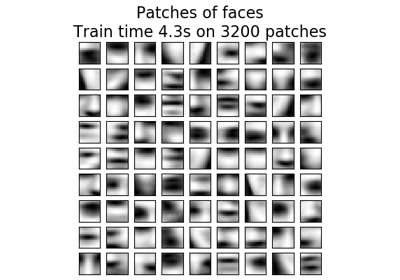Online learning of a dictionary of parts of faces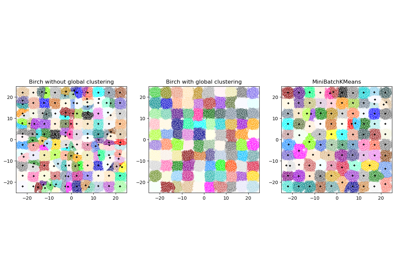Compare BIRCH and MiniBatchKMeans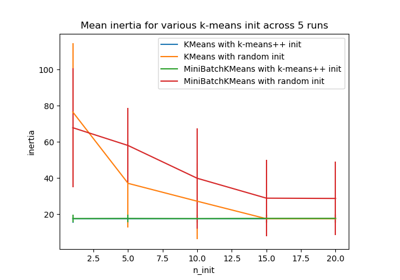Empirical evaluation of the impact of k-means initialization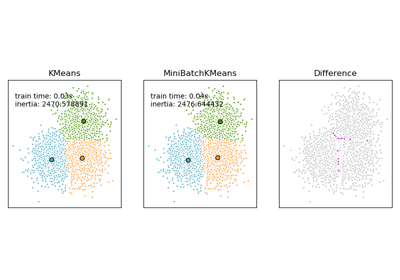Comparison of the K-Means and MiniBatchKMeans clustering algorithms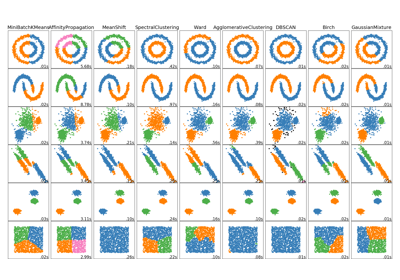Comparing different clustering algorithms on toy datasets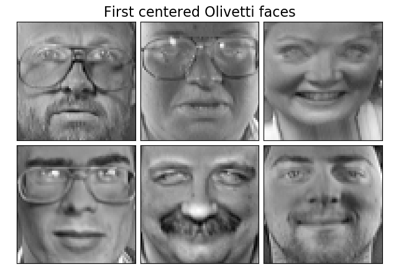Faces dataset decompositionsClustering text documents using k-means